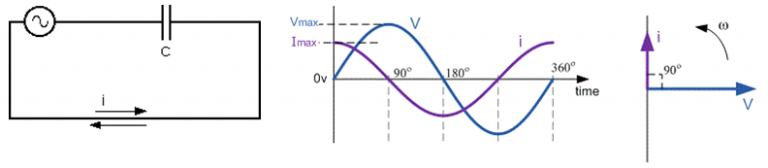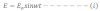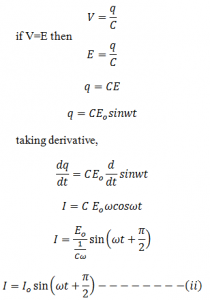# AC Through CapacitorLet an alternating emf be applied to a circuit containing capacitor only such type of circuit is called capacitive circuit.
Let the emf applied to the circuit is:Let q be the charge in the capacitor of capacitance C then the potential developed in the capacitor is:Equation ii is the type of current developed in the purely capacitive circuit.
Comparing equation i and ii we see that the alternating current leads to the alternating emf by π/2 as shown in fig.